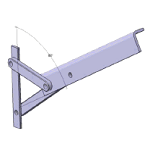# Geometric Logical MethodIntroduction to Geometric Logical Method The logical method is a structured geometric analysis problems of a geometric, as the name suggests. Allows orderly reasoning and evaluate a geometric problem, analyzing their determination and solution paths.

The geometric elements are defined by geometric constraints and values.

The way to evaluate the geometric form the basis of geometric logical method.

A structured view of the reasoning process and analysis of a geometric model can deal with problems in a scientific and structured.

The application of analysis methodologies makes practical sense as applied to problem solving. The proposed model is used as a strategic alternative to meet the exercises.

A collection of geometric problems, both directly and in engineering applications. Examples that model real elements to model geometrically teach everyday objects.